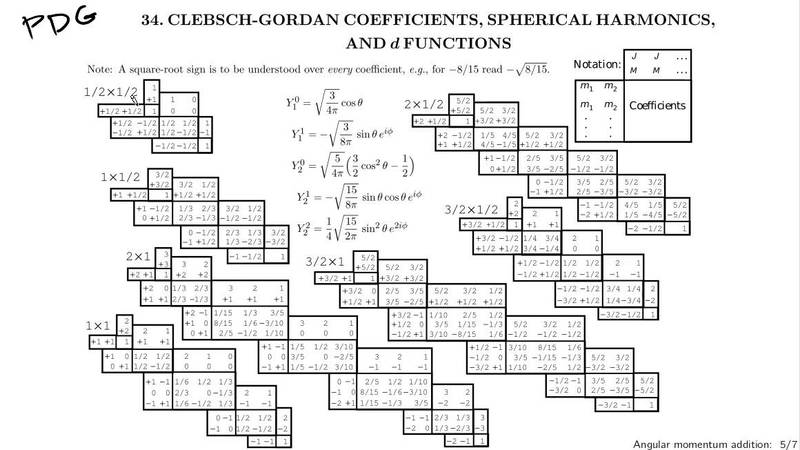# Total angular momentum state using two ways

• my physical romanace

#### my physical romanace

Consider addition of two angular momenta J = J1 + J2 , with j1=j2=1. Find the eigenstates of the total angular momentum I jm > in terms of the product states I j1 m1 j2 m2 > in two ways
(a) Make use of the tables of the Clebech _Gordan coefficients
(b) The state with m1 = m2 = 1 must be a state with j = m = 2 (why?). Apply J- repeatedly to this state to obtain all other states of j = 2. Form an appropriate linear combination of the two states with m1 + m2 =1 to obtain the state with j =1 , and m =1 . Find the other j = 1 states by applying J- repeatedly. Finally, find the j = m = 0 state by forming an appropriate linear combination of the three states with m1 + m2 = 0.
(c) Compare the results in (a) and (b).

I don't know how to use Clebech - Gordand coefficients, so please explain details using the table.

Welcome in PF!
Next time, please use the template when posting under homework section.That is the Clebsch-Gordan (CG) coefficient table for some values of ##j_1## and ##j_2##. The one you will be interested in is that in the bottom left denoted by (1x1). The way to read this table can be found in the upper right part of the picture. There you see that the composite state represented as ##|j,m\rangle## is specified by the pair of numbers in the upper part of each CG coefficient table. For example, the state ##|j=2,m=1\rangle## can be found in the second column. The two values ordered vertically below it: 1/2 and 1/2 tell you the coefficient of the state ##|j=2,m=1\rangle## when it is expanded in the ##|m_1\rangle |m_2\rangle## basis, after adding the square root. In this case you have
$$|j=2,m=1\rangle = \sqrt{\frac{1}{2}}|m_1=1\rangle |m_2=0\rangle + \sqrt{\frac{1}{2}}|m_1=0\rangle |m_2=1\rangle$$
or simply
$$|2,1\rangle = \sqrt{\frac{1}{2}}|1\rangle |0\rangle + \sqrt{\frac{1}{2}}|0\rangle |1\rangle$$

•my physical romanace